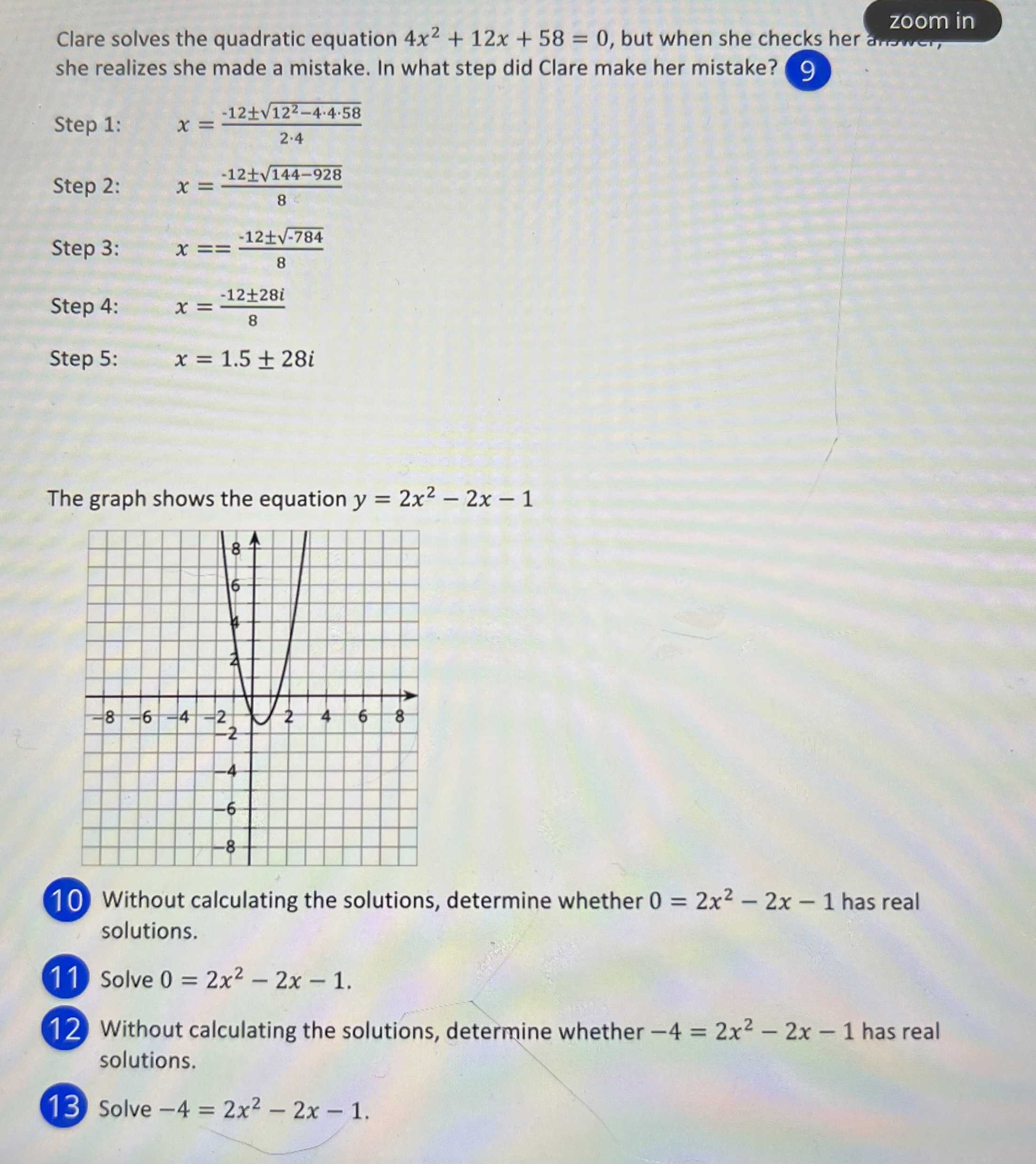### ¿Todavía tienes preguntas de matemáticas?

Pregunte a nuestros tutores expertos
Algebra
Pregunta10. Without calculating the solutions, determine whether $$0 = 2 x ^ { 2 } - 2 x - 1$$ has real solutions. (11) Solve $$0 = 2 x ^ { 2 } - 2 x - 1$$ . Without calculating the solutions, determine whether $$- 4 = 2 x ^ { 2 } - 2 x - 1$$ has real solutions.

$$12$$ Without calculating the solutions, determine whether $$- 4 = 2 x ^ { 2 } - 2 x - 1$$ has real solutions.

$$13$$ Solve $$- 4 = 2 x ^ { 2 } - 2 x - 1$$ .